NEET  >  Gravitational Potential Energy

# Gravitational Potential Energy Notes | Study Physics Class 11 - NEET

## Document Description: Gravitational Potential Energy for NEET 2022 is part of Physics Class 11 preparation. The notes and questions for Gravitational Potential Energy have been prepared according to the NEET exam syllabus. Information about Gravitational Potential Energy covers topics like What is Gravitational Potential Energy?, What is Gravitational Potential?, Gravitational Self Energy&nbsp;, Solved Problems and Gravitational Potential Energy Example, for NEET 2022 Exam. Find important definitions, questions, notes, meanings, examples, exercises and tests below for Gravitational Potential Energy.

Introduction of Gravitational Potential Energy in English is available as part of our Physics Class 11 for NEET & Gravitational Potential Energy in Hindi for Physics Class 11 course. Download more important topics related with notes, lectures and mock test series for NEET Exam by signing up for free. NEET: Gravitational Potential Energy Notes | Study Physics Class 11 - NEET
 Table of contents What is Gravitational Potential Energy? What is Gravitational Potential? Gravitational Self Energy Solved Problems
 1 Crore+ students have signed up on EduRev. Have you?

Gravitational potential energy is the energy possessed or acquired by an object due to a change in its position when it is present in a gravitational field. In simple terms, it can be said that gravitational potential energy is an energy that is related to gravitational force or to gravity.
The most common example that can help you understand the concept of gravitational potential energy is if you take two pencils. One is placed at the table and the other is held above the table. Now, we can state that the pencil which is high will have greater gravitational potential energy than the pencil that is at the table.
What we can learn here is that the pencil or any object, in particular, will have the potential to do work because of its location in the gravitational field. We will learn about the topic in detail below.

What is Gravitational Potential Energy?

When a body of mass (m) is moved from infinity to a point inside the gravitational influence of a source mass (M) without accelerating it, the amount of work done in displacing it into the source field is stored in the form of potential energy. This is known as gravitational potential energy. It is represented with the symbol Ug.
Explanation: We know that the potential energy of a body at a given position is defined as the energy stored in the body at that position. If the position of the body changes due to the application of external forces the change in potential energy is equal to the amount of work done on the body by the forces.
Under the action of gravitational force, the work done is independent of the path taken for a change in position so the force is a conservative force. Besides, all such forces have some potential in them.
The gravitational influence on a body at infinity is zero, therefore, potential energy is zero, which is called a reference point.

Gravitational Potential Energy Formula

The equation for gravitational potential energy is:
⇒ GPE = m⋅g⋅h
Where,

• m is the mass in kilograms
• g is the acceleration due to gravity (9.8 on Earth)
• h is the height above the ground in meters

Derivation of Gravitational Potential Energy Equation

Consider a source mass ‘M’ is placed at a point along the x-axis, initially, a test mass ‘m’ is at infinity. A small amount of work done in bringing it without acceleration through a very small distance (dx) is given by
dw = Fdx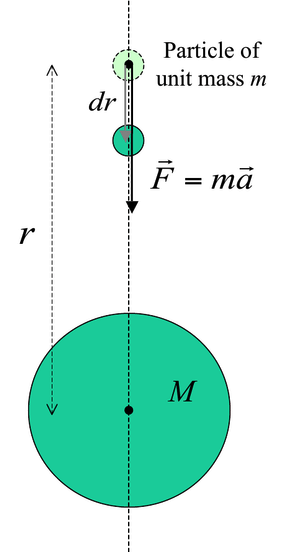Gravitational Potential

Here, F is an attractive force and the displacement is towards the negative x-axis direction so F and dx are in the same direction. Then,dw = (GMm/x2)dx
Integrating on both sides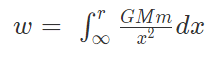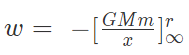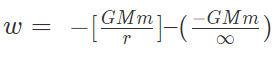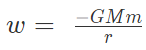Since the work done is stored as its potential energy U, therefore gravitational potential energy at a point which is at a distance ‘r’ from the source mass is given by;
U = -GMm/r
If a test mass moves from a point inside the gravitational field to the other point inside the same gravitational field of source mass, then the change in potential energy of the test mass is given by;
ΔU = GMm (1/ri – 1/rf)
If ri > rf then ΔU is negative.

Expression for Gravitational Potential Energy at Height (h) – Derive ΔU = mgh
If a body is taken from the surface of the earth to a point at a height ‘h’ above the surface of the earth, then r= R and r= R + h then,
ΔU = GMm [1/R – 1/(R+h)]
ΔU = GMmh/R(R + h)
When, h<<R, then, R + h = R and g = GM/R2.
On substituting this in the above equation we get,
Gravitational Potential Energy ΔU = mgh

Note:

• The weight of a body at the centre of the earth is zero due to the fact that the value of g at the centre of the earth is zero.
• At a point in the gravitational field where the gravitational potential energy is zero, the gravitational field is zero.

What is Gravitational Potential?

The amount of work done in moving a unit test mass from infinity into the gravitational influence of source mass is known as gravitational potential. Simply, it is the gravitational potential energy possessed by a unit test mass
⇒ V = U/m
⇒ V = -GM/r
Important Points:

• The gravitational potential at a point is always negative, V is maximum at infinity.
• The SI unit of gravitational potential is J/Kg.
• The dimensional formula is M0L2T-2.

Relation between Gravitational Field Intensity and Gravitational Potential

Integral Form: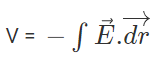(If E is given and V has to be found using this formula)
Differential Form:
E = -dV/dr (If V is given and E has to be found using this formula)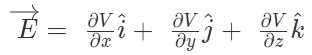(components along x, y and z directions).

Gravitational Potential of a Point Mass

Consider a point mass M, the gravitational potential at a distance ‘r’ from it is given by; V = – GM/r.

Gravitational Potential of a Spherical Shell

Consider a thin uniform spherical shell of the radius (R) and mass (M) situated in space. Now,

• Case 1: If point ‘P’ lies Inside the spherical shell (r<R):
As E = 0, V is a constant.
The value of gravitational potential is given by, V = -GM/R.
• Case 2: If point ‘P’ lies on the surface of the spherical shell (r=R):
On the surface of the earth, E = -GM/R2.
Using the relation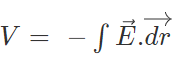over a limit of (0 to R) we get,
Gravitational Potential (V) = -GM/R.
• Case 3: If point ‘P’ lies outside the spherical shell (r>R):
Outside the spherical shell, E = -GM/r2.
Using the relation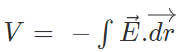over a limit of (0 to r) we get,
V = -GM/r.

Gravitational Potential of a Uniform Solid Sphere

Consider a thin uniform solid sphere of the radius (R) and mass (M) situated in space. Now,

• Case 1: If point ‘P’ lies Inside the uniform solid sphere (r < R):
Inside the uniform solid sphere, E = -GMr/R3.
Using the relation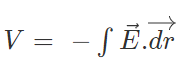over a limit of (0 to r).
The value of gravitational potential is given by,
V = -GM [(3R2 – r2)/2R2]
• Case 2: If point ‘P’ lies on the surface of the uniform solid sphere ( r = R ):
On the surface of a uniform solid sphere, E = -GM/R2. Using the relation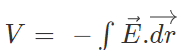over a limit of (0 to R) we get,
V = -GM/R.
• Case 3: If point ‘P’ lies outside the uniform solid sphere ( r> R):
Using the relation over a limit of (0 to r) we get, V = -GM/R.
• Case 4: Gravitational potential at the centre of the solid sphere is given by V =(-3/2) × (GM/R).

Gravitational Self Energy

The gravitational self-energy of a body is defined as the work done by an external agent in assembling the body from the infinitesimal elements that are initially at an infinite distance apart.

Gravitational Self Energy of a system of ‘n’ particles:

Let us consider n particle system in which particles interact with each other at an average distance ‘r’ due to their mutual gravitational attraction, there are n(n – 1)/2 such interactions and the potential energy of the system is equal to the sum of the potential energy of all pairs of particles i.e.,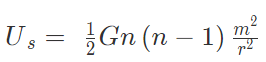Solved Problems

Example 1. Calculate the gravitational potential energy of a body of mass 10Kg and is 25m above the ground.
Solution: Given, Mass m = 10 Kg and Height h = 25 m
G.P.E is given as,
U = m × g × h = 10 Kg 9.8 m/s2 × 25 m = 2450 J.

Example 2. If the mass of the earth is 5.98 ×1024 Kg and the mass of the sun is 1.99 × 1030 Kg and the earth is 160 million Kms away from the sun. Calculate the GPE of the earth.
Solution: Given, the mass of the Earth (m) = 5.98 × 1024 Kg and mass of the Sun (M) = 1.99 × 1030 Kg
The gravitational potential energy is given by:
U = -GMm/r
U = (6.673 ∗ 10-11 ∗ 5.98 ∗ 1024 ∗1.99∗1030)/(160∗109) = 4963 x 1030 J

Example 3. A basketball weighing 2.2 kg falls off a building to the ground 50 m below. Calculate the gravitational potential energy of the ball when it arrives below.
Solution: GPE = (2.2 kg)(9.8 m/s2)(50 m) = 1078 J.

Example 4: A 2 kg body free falls from rest from a height of 12 m. Determine the work done by the force of gravity and the change in gravitational potential energy. Consider the acceleration due to gravity to be 10 m/s2.
Solution: Since, W = mgh
Substituting the values in the above equation, we get
W = 2 × 12 × 10 = 240 N
The change in gravitational potential energy is equal to the work done by gravity.
Therefore, Gravitational Potential Energy= 240 Joule.

The document Gravitational Potential Energy Notes | Study Physics Class 11 - NEET is a part of the NEET Course Physics Class 11.
All you need of NEET at this link: NEET

## Physics Class 11

126 videos|449 docs|210 tests
 Use Code STAYHOME200 and get INR 200 additional OFF

## Physics Class 11

126 videos|449 docs|210 tests

### How to Prepare for NEET

Read our guide to prepare for NEET which is created by Toppers & the best Teachers

Track your progress, build streaks, highlight & save important lessons and more!

,

,

,

,

,

,

,

,

,

,

,

,

,

,

,

,

,

,

,

,

,

;